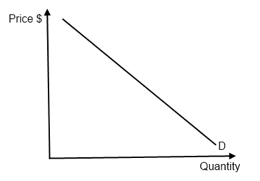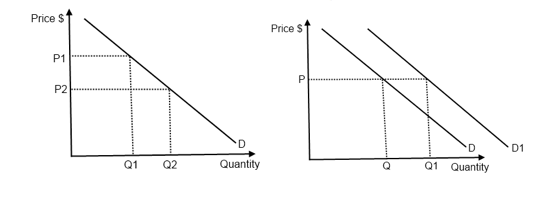# Demand

## The law of demand

Demand: is the total amount of goods and services that consumers are willing and able to purchase at a given price in a given time period.

The Law of Demand: states that "as the price of a product falls, the quantity demanded of the product will usually increase, ceteris paribus".

## The demand curve

Demand curve: represents the relationship between the price and the quantity demanded of a product, ceteris paribus.Figure 1.1 - A demand curve

## The non-price determinants of demand (factors that change demand or shift the demand curve)

The non-price determinants of demand (shifting) for normal & inferior goods:

1. Income: As income rises
• demand for normal goods rises, demand curve shifts to the right
• demand for inferior goods falls, demand curve shifts to the left (when income gets to certain level, demand will become zero and so the demand curve disappears)
2. Taste & Preferences: Change in tastes in favor (i.e. advertising campaign) demand curve shifts to the right
3. Price of substitutes and complements:
• As price of substitutes increases (movement along the curve) the demand shifts to the right
• As price of complements increases (movement along the curve) the demand shifts to the left
4. Demographic changes: if population grows, the demand for most products will increase, thus the demand curves shift to the right?more will be demanded at each price level

## Movements along and shifts of the demand curve

Movement along the demand curve:

• A change in price of the good itself leads to a movement along the existing demand curve (price is the axes), while a change in any other determinants of demand will always lead to a shift of the demand curve to either left or to the right.Figure 1.2 - Movement along and a shift of the demand curve

## Linear demand functions (equations), demand schedules and graphs

Linear demand functions:

Where:

• a = quantity demanded when price is zero
• b = slope of the curve
 Price \$ Calculation Quantity demanded 0 60 1 55 2 50

If:

• a changes → shift of demand curve
• b changes → change of steepness of demand curve